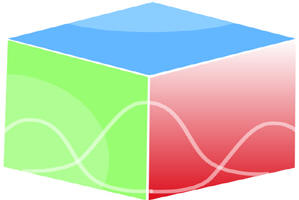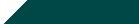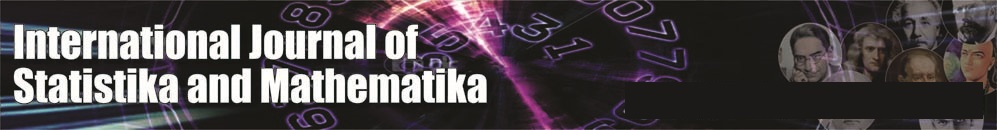``Home| Journals | Statistics Online Expert | About Us | Contact UsUntitled Document

On Certain Conditions of Geometric Functions for the Generalized Hypergeometric Functions

Chena Ram1 and Garima Agarwal2

2Research Scholar, Department of Mathematics and Statistics, Jai Narain Vyas University, Jodhpur, Rajasthan, INDIA.

Research Article

Abstract: The aim of the present paper is to obtain sufficient conditions for the function  3R2( for its belongingness to certain subclasses of starlike and convex functions. Similar results, using integral operator, are also obtained.

Key words: Starlike Functions, Convex Functions, Hypergeometric Functions, Clausenian Hypergeometric functions, Integral operator.

1. Introduction

Let   denote the class consisting of functions  given by

(1.1)

which are analytic and univalent in the open unit disk U = {

Let  is the subclass of the class  Which has functions satisfying the condition

(1.2)

for some  and for all

Also let  denote the subclass of  constituted by functions those satisfy the condition

(1.3)

for some  and for all

From (1.2) and (1.3), we have

.                                                                   (1.4)

We note that   the class of starlike functions of order

and , the class of convex functions of order                       (see Silverman).

Following definitions  will be required in proving the main results:

33R2(               (1.5)

where
If we set , then the generalized hypergeometric function 3 reduces to the result due to Virchenko, Kalla and Zamel , as

22R1(.                                         (1.6)

For  (1.5) reduces to the Clausenian hypergeometric function studied by Saxena and Kalla 

3F2(,                                                              (1.7)

and for , (1.6) reduces to the Gauss hypergeometric function

2F1(                                                                             (1.8)

In the present paper we shall use the following lemmas, see Altintas and Owa .

Lemma 1. A function  of the form (1.1) is in the class , if and only if

) (1.9)

Lemma 2. A function  of the form (1.1) is in the class , if and only if

)                                                            (1.10)

Main Results

Theorem 2.1. If   then  3R2( is in the class , if and only if

3R2(

3R2(            (2.1)

Proof: Since

3R2(

,              (2.2)

by Lemma 1, it is sufficient to prove that

)
Now we have

)

(1

i.e.           (1

i.e            (1                                                                                                                                      +,

which on using (1.5) yields

3R2(

3R2(

The theorem is completely proved.

Corollary 1.   3F2( is in the class , if and only if

3F2(

3F2( (2.3)

Proof. If we set =1 in (2.1), the proof is completed.

Corollary 2.   2R1( is in the class , if and only if

2R1(

2R1(  (2.3)

Proof. If we set  in (2.1), the proof is completed.

Theorem 2.2. If  ), then

R2(  [2 − 3R2( is in the class , if and only if

3R2(

3R2(                  (2.5)

Proof.

R2(          (2.6)

By Lemma 1, we need only to show that

Now,

=

i.e.          =

=

Hence,

3R2(

3R2( .

The proof is completed.

Corollary 3. F2(  [2- 3F2( is in the class  if and only if

3F2(

3F2(                                                  (2.7)

Proof. If we set  in (2.5), the proof is completed.

Corollary 4. R1(  [2- 2R1( is in the class  if and only if

2R1(

2R1(                                       (2.8)

Proof. If we set  in (2.5), the proof is completed.

Theorem 2.3. If   then  3R2( is in the class , if and only if

3R2(

3R2(

3R2(                             (2.9)Proof. From (2.2), we have

3R2(

By Lemma 2, it is sufficient to prove that

)

Now we have

+

++

+

+

+

+

+

=3R2(

3R2(   3R2(

The proof is completed.